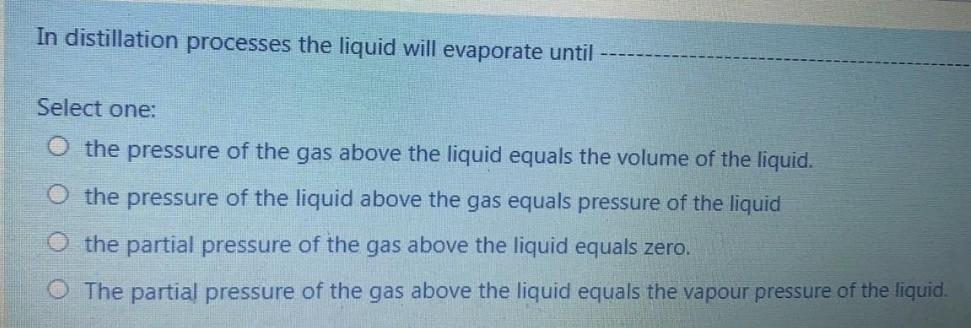### Create an Account

Home / Questions / In distillation processes the liquid will evaporate until Select one: the pressure of the ...

# In distillation processes the liquid will evaporate until Select one: the pressure of the gas above the liquid equals the volume of the liquid. the pressure of the liquid above the gas equals pressure

In distillation processes the liquid will evaporate until Select one: the pressure of the gas above the liquid equals the volume of the liquid. the pressure of the liquid above the gas equals pressure of the liquid the partial pressure of the gas above the liquid equals zero. The partial pressure of the gas above the liquid equals the vapour pressure of the liquid.May 17 2021 View more View LessSubscribe To Get Solution